Perpendicular and Parallel Lines
edHelper subscribers - Create a new printable

Number of Keys
 Select the number of different printables: 1 key 2 keys 3 keys 4 keys 5 keys

Number of Pages (for each key)
 Select the number of pages: 1 page 2 pages 3 pages 4 pages

Answer Key
Include an answer key (answer keys will be at the end of the printable)
No answer key
Not a subscriber?  Sign up now for the subscriber materials!
High School Geometry
High School Geometry
Math

(pre-made sample)
 Name _____________________________Date ___________________ (Key 1 - Answer ID # 0371087)
1.

Two skew lines are ______ parallel.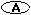never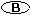always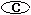sometimes

2.

Two parallel lines are ______ coplanar.neveralwayssometimes

3.

Quadrilateral PQRS is a parallelogram. If m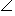5 = 64, then m1 + m2 + m3 + m4 = ______.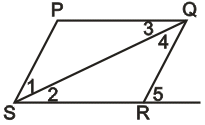64128192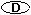Impossible to tell

4.

If k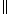n, then m1 + m2 + m3 + m4 = ______.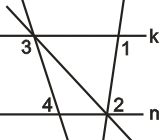901803602705.

kln and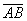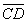. If m1 = 79, m2 = 66, and m3 = 32, then mx + my + mz = ______.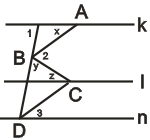6.

Two lines perpendicular to a plane are ______ skew lines.neveralwayssometimes

Perpendicular and Parallel Lines
edHelper subscribers - Create a new printable

Number of Keys
 Select the number of different printables: 1 key 2 keys 3 keys 4 keys 5 keys

Number of Pages (for each key)
 Select the number of pages: 1 page 2 pages 3 pages 4 pages

Answer Key
Include an answer key (answer keys will be at the end of the printable)
No answer key
Not a subscriber?  Sign up now for the subscriber materials!
High School Geometry
High School Geometry
Math